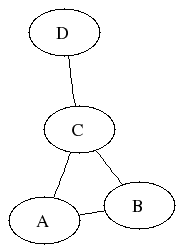Graph TheoryA simple graph with labelled vertices
Graph Theory is an area of Pure Maths that investigates and studies the way things can be connected.

It all started with Leonhard Euler and the Bridges of Koenigsberg problem. In that problem the only thing that mattered was the pieces of land that were connected. The exact shape, placing and lengths of the bridges were irrelevant.

Graph Theory is also the area with one of the most common examples of Pure Maths found in school, namely, the Four Colour Theorem.

 Given any map, colour the regions so that regions sharing a border get different colours. How many colours do you need?

This question was first posed in 1852, and it wasn't until 1976 that a proof was finally given. Even then there was, and still is, some controversy, because the proof requires a computer to check a large number of sub-cases. These then have to be combined in a clever way - the computer doesn't actually do the proof - but even so, it's not a proof in the traditional sense.

You can read more about the Four Colour Theorem and Graph Theory in general here:

Another problem that turns out to be an example in graph theory is on the Dominoes Unlimited page.
On this page, if anyone asks, I'll discuss some of the problems and challenges in Graph Theory, the interesting and unexpected places it's been used, and some of the basic theory.

ContentsSuggest a change ( <-- What does this mean?) / Send me email